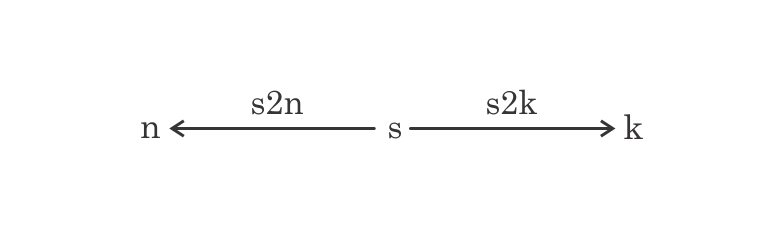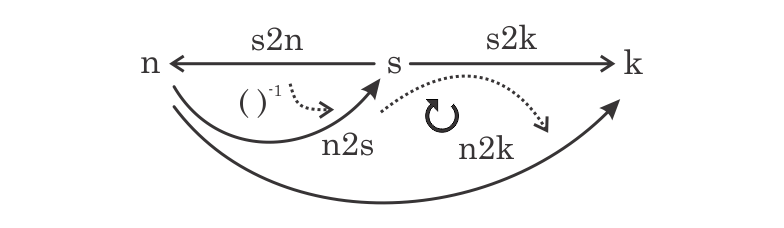# Intro To Categorical Programming

### Have You Shorts Or Opens?

Categorical programming was applied to the building of the Clipper microprocessor.  Read how part of it was done.

Beginning work ’84 at Fairchild Semiconductor as a CAD engineer on the Clipper microprocessor, one of the early assignments was to look at a pen plotter.  A pen plotter possesses a few ink cartridges in a carousel, and if they possess ink, you drive the plotter to pick up a pen from the carousel, move off to draw a rectangle, text, or some such by ‘pen up’ and ‘pen down’ commands.  Eventually your circuit appears (if ink holds out).

Designing the Clipper microprocessor involved drawing shapes by layout designers on a number of layers.  Some layers are for the transistors, and some are for metal layers above that wire the transistors underneath.  How many layers depends on the complexity of the process building your chips.

A circuit designer has an assigned layout designer drawing on Applicons (later Calmas).  The circuit designer is salary while the layout designer is hourly.  The circuit designer does simulations to determine sizes of critical features for the layout designer to draw.  The layout designer, aka polygon pusher, draws the transistors and metal connections according to those specs.  Eventually the layout designer generates a picture of the circuit, and in those early days, turns to the pen plotter.

The plotter attaches to an VAX VMS-780 through a serial line dangling from the ceiling.  Somehow a GDS file from the Applicon is submitted to the plotter, drawing shapes particularly tediously as a layer change mandates a trip to the pen carousel to fetch a different color.  The probable drawn shape order being the layout designer’s drawing sequence.  For a pen plotter watcher, this is gruesome.

So write code to bin the shapes into files of given layers.  Writing these binned layers to the pen plotter has no pen change until the entire layer of shapes finishes.  These containers of shapes have an index for each shape.  This allows representations in the form s→geo2 where geo2 is the space of flat 2D polygons, and s is a finite set.  From here we bin/layer/index neighboring constructs to connect shapes, nets, inputs, outputs, and so on in a way to get a meaningful machine.

When a building a chip, a net list describes the wiring connections between the transistors.  The nets in the net list become wires in the chip during the design process.

Typically when building a chip, we often know which shapes belong to which nets as that is how they were constructed. Letting n denote nets and s shapes as above, we have the shape-to-net mapping s2n: s→n.

But things can go wrong during construction, and two significant issues for any electrical circuit are shorts and opens.  A short occurs when two nets touch each other, like power and ground.  A power-ground short is really bad, but any any short between any two nets, be it signal, power, clock et al, is bad.  An open is a net that is not finished during the design process and is electrically incomplete.  Either someone or something forgot to put in a connection, or a block of circuits moved in such a way on the chip that it no longer properly connects, or some other variety of catastrophe.

Each construction phase in a chip has a validation phase following.  Once we have our nets and then our shapes generated by layout designers and automated tools (placers, routers, …), electrical connectivity is determined by what shapes touch which other ones.  Touching allows for shapes on the same layer, or touching those representing holes in an insulation layer so as to connect vertically.  Such a tool is called an extractor, and it determines the electrical nodes for all the shapes.  This gives the shape-to-node mapping s2k: s→k where k represents the nodes that have been found.  Pictorially we have the following diagram.

 n Nets s Shapes k Electrical nodesWe generally refer to the arrows as morphisms or maps.  You can think of them as functions.  Take note, we’ll be given to use partially defined functions for their convenience in our diagrams.

Now somehow compare the nets to the nodes.  Ideally, nets and electrical nodes are the same thing, and that is an isomorphism, here meaning the sets’ elements are in one-to-one correspondence.  To do so we need to determine the deviation from the ideal.  We introduce an operator, inversion, on a morphism such as s2n.  Since s2n is a function, the inverse of s2n is a partial function from nets n to shapes s.  Namely, each net is mapped to the set of all shapes that are assigned to that net.  Effectively this new function n2s has as its domain, its starting set, n and its codomain, the ending set, as the set of all subsets of shapes s.  Sometimes the set of all subsets of some set X is called the power set  P(X) of X.  Denote this as 2X, the subsets of X, since the functions from X to 2 = {true,false} give the subsets of X, namely that portion of the function on which it is true.  To be general we use ΩX where Ω denotes the available truth values in our math system as perhaps later we want more than two.

Normally one writes such an inverse as n2s: n→2s.  Instead we use a convention of replacing the “open headed” arrow with a “heavy headed” arrow and show the codomain as s instead of 2s, the subsets of s.  The dotted arrow represents the action flow showing the inverse operator ()-1 inverting s2n:By taking the composite of n2s followed by s2k we get a mapping of nets n to electrical nodes k.  Of course, this composite n2k maps to codomain 2k, the subsets of k, as it takes a net to the electrical nodes it connects to through the shapes s.  So one net mapping to, say, two electrical nodes means that net has an open.  The next diagram has a circular arrow representing that cell of the diagram is commutative, meaning that both ways around it are equal.  In this case that is due to the composition of n2s with s2k to define n2k:Similarly, if one works first with s2k and works back to nets n, then one obtains a mapping from electrical nodes to sets of nets.  That is, if one electrical nodes maps through the shapes to more than one net, then those nets are shorted together.

Though we may have at this point the functions n2k and k2n that embed inside them the shorts and opens we are looking for, we may need to extract that information to make it useful, such as a list of which nets are open and which electrical nodes represent shorts.  This information is then used for debugging the chip.

Call the function card for cardinality that takes a set from 2n and maps it to the number of elements in that set.  It has the form card:2n→N where N denotes the natural numbers {0, 1, 2, …}.  We could also imagine it has the form card:n→N where each element is sent to 1.  Another useful function is “greater than one” >1: N→Ω which returns true for any natural number greater than one, and false otherwise.  At this stage the following composite nIsOpn takes each net to the number of electrical nodes its shapes are on.As Ω={true,false}, the nIsOpn map sends all those nets to true that are open.  That specifies a subset of n that are the opens, and we can use the pullback along the sub-object classifier true:1→Ω to get the open nets nopen in n.  A pullback between two morphisms to the same codomain can be thought of as the best common elements between the two.By first working with s2k, inverting it, and proceeding similarly as above, we obtain a subset kshort of k giving those electrical nodes representing shorts.  The ∃! represents the one and only possible full mapping to the terminal object (a one point set).  We use the notion “full” here as for a terminal object in a respectable category there exists a unique (that’s the ∃!) morphism to 1, but for a partial functions there could be many, but only one “full”.

Now that our computation is done, a few items to note:

There are no “if” statements.  One could very well write an “if” statement if one likes in a diagram (a later post), but the above computation determining shorts and opens makes no evident use of a classic “if” statement.

The computation is not rigid in the sense that one must first have s2n and s2k first before any “next” computation is done.  Partial information, as it comes in for defining both s2n and s2k, can be fed into the diagram.  Basically the diagram above exposes parallelism in the computation, from the inverting of maps, to compositions, to pullbacks, and so on.

There are no loops in the sense of for’s or while’s pervading traditional computing.  Perhaps in the implementation of such features there are said details, but at the actual level of intent there’s no need of their direct use.

There is some sloppiness in the diagram above, though, as compared to purists’ customs.  One is the use of partial maps.  Another is the “heavy headed” arrow and confusing the difference between objects k and 2k, the subsets of k, as the compositions are taken.  Nonetheless, these shorthands are useful in keeping the size and tedium of the diagrams at hand.

In the language developed at the time the diagram executes in precisely three commands:

• Invert s2n to n2s.
• Compose n2s with s2k to get n2k.
• Classify those n as opens whose cardinality of its k’s is greater than one.

Given the nature of the derivation as a commutative diagram, or proof, the program executes correctly, and quickly, the first time.

Check out the next in this series:  V Is For Vortex – More Categorical Programming